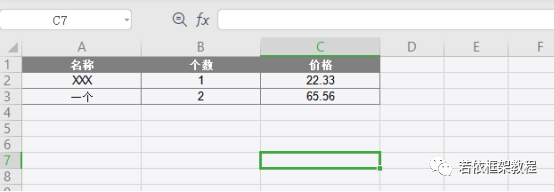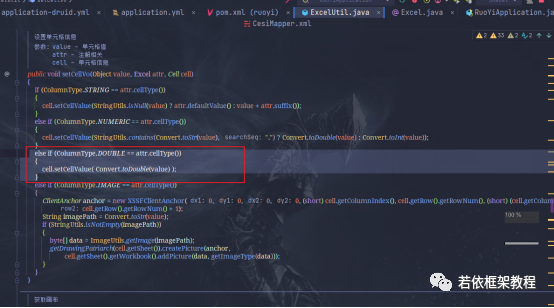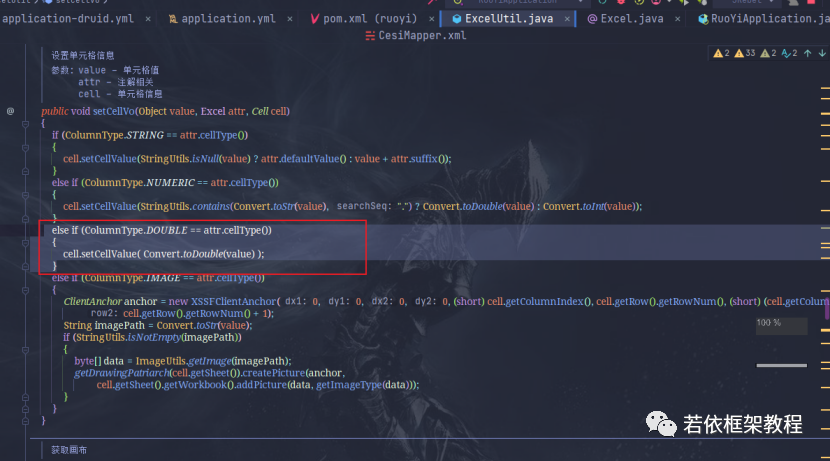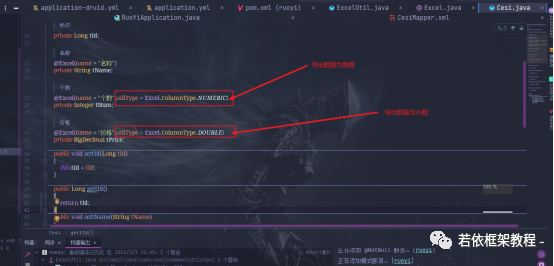# RUOYI 框架教程 10 |若依 Excell 数据导出小数处理，你会么！Java_若依框架教程若依教程独家分享！点赞+关注，一起从「蒟蒻」变成「大佬」~

# 若依数据导出小数处理，你用过么？

## 本期内容# 2 代码应该怎么调？

## 本期内容

1，修改 Excell.java 工具类的 ColumnType 方法，完善代码，加入更多数据类型，包括：NUMERIC(0) 整数 ，STRING(1) 文本， IMAGE(2)  图片， DOUBLE(3) 小数

 public enum ColumnType    {        NUMERIC(0), STRING(1), IMAGE(2),DOUBLE(3);        private final int value;        ColumnType(int value)        {            this.value = value;        }        public int value()        {            return this.value;        }    }2，修改 ExcellUtil.java 工具类的 setCellVo 方法，完善代码，添加下面代码：

else if (ColumnType.DOUBLE == attr.cellType()){    cell.setCellValue( Convert.toDouble(value) ); }

public void setCellVo(Object value, Excel attr, Cell cell)    {        if (ColumnType.STRING == attr.cellType())        {            cell.setCellValue(StringUtils.isNull(value) ? attr.defaultValue() : value + attr.suffix());        }        else if (ColumnType.NUMERIC == attr.cellType())        {            cell.setCellValue(StringUtils.contains(Convert.toStr(value), ".") ? Convert.toDouble(value) : Convert.toInt(value));        }        else if (ColumnType.DOUBLE == attr.cellType())        {            cell.setCellValue( Convert.toDouble(value) );        }        else if (ColumnType.IMAGE == attr.cellType())        {            ClientAnchor anchor = new XSSFClientAnchor(0, 0, 0, 0, (short) cell.getColumnIndex(), cell.getRow().getRowNum(), (short) (cell.getColumnIndex() + 1),                    cell.getRow().getRowNum() + 1);            String imagePath = Convert.toStr(value);            if (StringUtils.isNotEmpty(imagePath))            {                byte[] data = ImageUtils.getImage(imagePath);                getDrawingPatriarch(cell.getSheet()).createPicture(anchor,                        cell.getSheet().getWorkbook().addPicture(data, getImageType(data)));            }        }    }3，修改 Cesi.java 实体类代码，加入：

cellType = Excel.ColumnType.NUMERIC 导出为整数及小数cellType = Excel.ColumnType.NUMERIC 导出为整数及小数## 评论